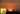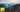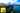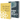# Improving Gradient Descent in JavaScript

by Robin WieruchA recent article gave an introduction to the field of machine learning in JavaScript by in a univariate regression problem. There are several improvements to optimize the gradient descent algorithm that should be collected in this article.

In general, when dealing with a regression problem, the outcome can already be influenced by . It is not often the case that data points correlate linear to each other. Hence the correlation is often curvilinear and thus it could be a polynomial regression instead of a linear regression. But that isn't specific to gradient descent. So let's dive into the properties to improve gradient descent.

I highly recommend to take the Machine Learning course by Andrew Ng. This article will not explain the machine learning algorithms in detail, but only demonstrate their usage in JavaScript. The course on the other hand goes into detail and explains these algorithms in an amazing quality. At this point in time of writing the article, I learn about the topic myself and try to internalize my learnings by writing about them and applying them in JavaScript. If you find any parts for improvements, please reach out in the comments or create a Issue/Pull Request on GitHub.

## Learning Rate in Gradient Descent

The gradient descent algorithm converges with a multitude of iterations to a local minimum (which could be the global minimum as well). The learning rate alpha determines how fast the gradient descent algorithm converges. But you cannot simply choose a high learning rate.

The learning rate alpha is crucial for gradient descent to succeed. If the learning rate alpha is chosen too low, it takes ages until the algorithm will converge to a minimum. It takes mini steps downhill to find the valley rather than taking a risk to accelerate the algorithm. However, if the learning rate alpha is chosen too high, it be possible that gradient descent never reaches the minimum, because it surpasses it by taking too big steps. It can happen that one step already ends up on the next hill and missed the valley in between. So how to choose the correct learning rate?

Fortunately you have the cost function. The returned cost of the function should be minimized by every step the gradient descent algorithm takes towards a minimum. Thus you can observe the cost while gradient descent is performed. The cost should decrease. If they increase, your learning rate is too high and you need to decrease it. You can start out with different learning rates (0.01, 0.001, 0.0001, 0.03, 0.003, 0.0003, ...) in the beginning and adjust them over time depending on your performance of the cost function.

Check out the gradient descent algorithm implementations and tweak their learning rates to get a feeling for them. It is often a fine balance between a too high and too low learning rate.

## When does gradient descent converge?

Gradient descent converges eventually when selecting an appropriate learning rate. But when should you decide to stop it, because the algorithm is trained to make predictions? Basically there are two ways to stop it.

The obvious one is to stop it after a certain number of iterations (e.g. 1500). However, this way you never know if gradient descent converged to a minimum. You could have messed up the learning rate in the first place and thus your cost function wouldn't have minimized the returned cost even though the algorithm ran with a high number of iterations. You would have to observe the returned cost manually when running the algorithm.

There is a more sophisticated way: Stop it when the cost decreases by less than a given amount (threshold) for one iteration. That's the point where the cost reached a certain minimum and thus the gradient descent algorithm is trained. If the learning rate is messed up in the first place, the algorithm might never stop, because the threshold doesn't go below the defined amount. Then there could be an escape condition to stop the algorithm after a certain very high number of iterations. Afterward, the algorithm should tell that it didn't converge but only ran all iterations and you should start over with a different learning rate.

## Choosing Initial Parameters in Gradient Descent

The parameters in a hypothesis function are trained by using gradient descent. For instance, a hypothesis function for a simple univariate linear regression is defined as `h(x) => thetaZero + thetaOne * x`. The input value x (e.g. size of house) stays fixed the same as the output value y (e.g. price of a house) in a prediction (hypothesis). But the parameters theta are trained over the course of the training algorithm.

When starting out with gradient descent, you have to choose the theta parameters randomly. Most often, these parameters start out with the value 0. As you know, a gradient descent algorithm converges eventually towards a minimum. But the minimum doesn't have to be a global minimum. In a multivariate regression problem it can be a local minimum, because there are multiple local minima and only one global minimum. Depending on the initial parameters for theta, you may end up in different local minima but never in the global minimum. Thus you have to try different initial parameters for theta in order to find out about different local minima and perhaps the global minimum. Thus another optimization for gradient descent is choosing different initial parameters for theta to start out at different locations in the hilly landscape to find out about different valleys.

## Feature Scaling for Gradient Descent

In a multivariate training set, not all features share the same range. For instance, number of bedrooms of a house may range from 1 to 10 whereas the size of a house goes up to the thousands. Therefore, it becomes difficult for the gradient descent algorithm to adjust the parameters for the hypothesis function when there is such a gap between the features. The algorithm is way more efficient when the features roughly share the same range. It is because because the parameters will adjust quickly on small ranges and slowly on large ranges. When the variables of the features are uneven, it becomes an inefficient task to descend towards the minimum.

The optional range is between -1 and 1 for each variable of a feature. But it isn't a strict requirement, since it should only help to improve gradient descent. There are different methods for feature scaling:

• Mean normalisation
• Standardization
• Rescaling
• ...

For instance, in a mean normalization the feature scaling can be performed with `xScaled = (x - mean(x)) / (max(x) - min(x))`. So if x represents number of bedrooms with a range of 3 to 5 and a mean value of 4, then `x = (bedrooms - 4) / 2`.

If you want to experience how feature scaling is implemented in JavaScript by using the standardization method, checkout the implementation of a where feature scaling becomes necessary.

## Vectorization in Machine Learning and JavaScript

A first naive implementation of gradient descent often involves programmatic loops. The algorithm needs to run through a lot of iterations to learn (which it still needs to after the vectorization), but also through all the data (m times) in the training set. In a multivariate linear regression, the algorithm has to run through all the features as well (n times). It becomes an inefficient computation and tedious task to implement eventually when using programmatic loops. That's where .

By using a vectorized implementation, gradient descent can be expressed as simple as

`for (let i = 0; i < ITERATIONS; i++) {  theta = theta - ALPHA / m * ((X * theta - y)' * X)'}`

rather than

`for (let i = 0; i < ITERATIONS; i++) {  for (let j = 0; j < m; j++) {    thetaZeroSum += hypothesis(x[j]) - y[j];    thetaOneSum += (hypothesis(x[j]) - y[j]) * x[j];  }   thetaZero = thetaZero - (ALPHA / m) * thetaZeroSum;  thetaOne = thetaOne - (ALPHA / m) * thetaOneSum;}`

The for loop for the iterations itself stays, but the computation of theta becomes a one liner by using a vectorized implementation. Here you can find the . Only by mastering the matrix operations in your programming language, it becomes efficient to apply machine learning algorithms.

## Alternative: Normal Equation

In a regression problem there is an alternative to gradient descent which is called normal equation. It doesn't need any iterative process to reduce the cost function. By explicitly taking the derivates, the function finds the optimum parameters for theta. The mathematical expression for a normal equation is `inv(X' * X) * X' * y`. You can read more about it in this article about the , because it isn't superior to gradient descent and should only be used when it makes sense.

The list of improvements for gradient descent may not be complete, because I myself just started out to learn about machine learning. Do you have any more improvements for gradient descent? Please leave your comments below.## Gradient Descent with Vectorization in JavaScript

A recent article gave an introduction to the field of machine learning in JavaScript by predicting housing prices with gradient descent in a univariate regression problem. It used plain mathematical…## Linear Regression with Normal Equation in JavaScript

A recent article gave an introduction to the field of machine learning in JavaScript by predicting housing prices with gradient descent in a univariate regression problem. It used plain mathematical…